UPSC  >  Important Notes: Quadratic & Higher Order Equations

# Quadratic & Higher Order Equations Important Notes - CSAT Preparation - UPSC

 Table of contentsTheory of EquationsQuadratic EquationsImportant Formulas for Solving Quadratic EquationsQuadratic Equation: Roots, Nature of Roots & CoefficientHow to Solve Quadratic Equations?Cubic EquationHigher Degree Equations/ Biquadratic EquationQuadratic Equations form a fundamental part of competitive exams, testing candidates' mathematical reasoning and problem-solving abilities. These equations often serve as a bridge between basic algebraic concepts and more advanced mathematical applications, making them a crucial foundation for various topics. When preparing for Quadratic Equations, focus on fundamental concepts. Remember that quadratic equations have two roots, and these roots can be either real or imaginary. Having a good grasp of these basic concepts will help you solve questions more efficiently.

## Theory of Equations

The equation is a valid expression with an equal sign (=), representing the balancing of the given terms on both sides of it. Solving these equations, we may get some root values of the unknown variables given in it.

1. Linear Equation Formula

• A linear equation is an equation that can be written in the form given as: ax + b = 0
• Where a and b are the real numbers and x is a variable. This form is popular as the standard form of a linear equation. Also, the variable may or may not be x, so don’t try to identify only this as variable. Its only one root say ‘p’ will be possible, which will be as: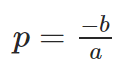• A quadratic equation is an equation having a second degree of the variable. It means it contains at least one term which is squared. The standard form of the quadratic equation is:
ax2+bx+c=0,
with a, b, and c being constants or the coefficients, and x is the variable, which we need to evaluate. One absolute rule is that the coefficient “a” must be non-zero.
• The quadratic equation is one of the popular concepts of algebra. By using the equations, this quadratic form can be solved and solutions will be resolved. If the roots of the quadratic equation are p and q, then we have the following formulas: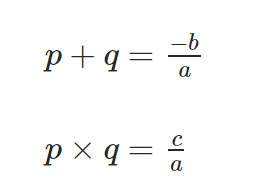3. Cubic Equation Formula

• A cubic equation is an equation which is having the highest degree of the variable term as 3. Its standard form is given as:
ax3+bx2+cx+d=0
where a,b,c,d are the coefficients, with the condition that coefficient “a” must be non-zero.
• According to the fundamental theorem of algebra, the cubic equation will always have 3 roots. Some or all of the roots may be equal also. Let the three roots of the cubic equation are p, q, and r, then the following formula will hold: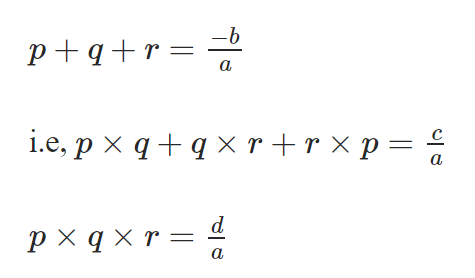Quadratic equations are the polynomial equations of degree 2 in one variable of type f(x) = ax2 + bx + c where a, b, c, ∈ R and a ≠ 0.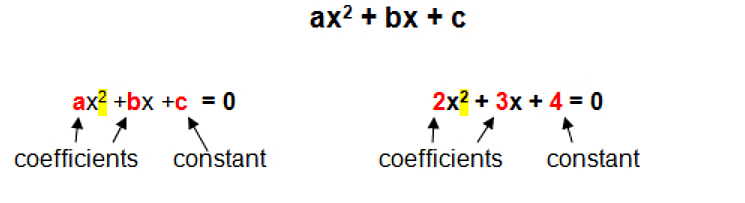• It is the general form of a quadratic equation where ‘a’ is called the leading coefficient and ‘c’ is called the absolute term of f (x). The values of x satisfying the quadratic equation are the roots of the quadratic equation (α, β).
• The quadratic equation will always have two roots. The nature of roots may be either real or imaginary.
• A quadratic polynomial, when equated to zero, becomes a quadratic equation. The values of x satisfying the equation are called the roots of the quadratic equation.
• General from: ax2 + bx + c = 0
Examples: 3x2 + x + 5 = 0, -x2 + 7x + 5 = 0, x2 + x = 0.

## Important Formulas for Solving Quadratic Equations

1. The roots of the quadratic equation: x = (-b ± √D)/2a, where D = b2 – 4ac
2. Nature of roots:

• D > 0, roots are real and distinct (unequal)
• D = 0, roots are real and equal (coincident)
• D < 0, roots are imaginary and unequal

3. The roots (α + iβ), (α – iβ) are the conjugate pair of each other.
4. Sum and Product of roots: If α and β are the roots of a quadratic equation, then

• S = α+β= -b/a = coefficient of x/coefficient of x2
• P = αβ = c/a = constant term/coefficient of x2

5. Quadratic equation in the form of roots: x2 – (α+β)x + (αβ) = 0
6. The quadratic equations a1x2 + b1x + c1 = 0 and a2x2 + b2x + c2 = 0 have;

• One common root if (b1c2 – b2c1)/(c1a2 – c2a1) = (c1a2 – c2a1)/(a1b2 – a2b1)
• Both roots common if a1/a2 = b1/b2 = c1/c2

7. In quadratic equation ax+ bx + c = 0 or [(x + b/2a)2 – D/4a2]

• If a > 0, minimum value = 4ac – b2/4a at x = -b/2a.
• If a < 0, maximum value 4ac – b2/4a at x= -b/2a.

8. If α, β, γ are roots of cubic equation ax3 + bx2 + cx + d = 0, then, α + β + γ = -b/a, αβ + βγ + λα = c/a, and αβγ = -d/a
9. A quadratic equation becomes an identity (a, b, c = 0) if the equation is satisfied by more than two numbers i.e. having more than two roots or solutions either real or complex.

Question for Important Notes: Quadratic & Higher Order Equations
Try yourself:What are the roots of the equation x2 - 9x - 10?

## Quadratic Equation: Roots, Nature of Roots & Coefficient

### 1. Roots of a Quadratic Equation

The solution or roots of a quadratic equation are given by the quadratic formula:
(α, β) = [-b ± √(b2 – 4ac)]/2ac
• The values of variables satisfying the given quadratic equation are called its roots. In other words, x = α is a root of the quadratic equation f(x), if f(α) = 0.
• The real roots of an equation f(x) = 0 are the x-coordinates of the points where the curve y = f(x) intersect the x-axis.
• One of the roots of the quadratic equation is zero and the other is -b/a if c = 0
• Both the roots are zero if b = c = 0
• The roots are reciprocal to each other if a = c
• Discriminant: The term (b2 – 4ac) in the quadratic formula is known as the discriminant of a quadratic equation. The discriminant of a quadratic equation reveals the nature of roots.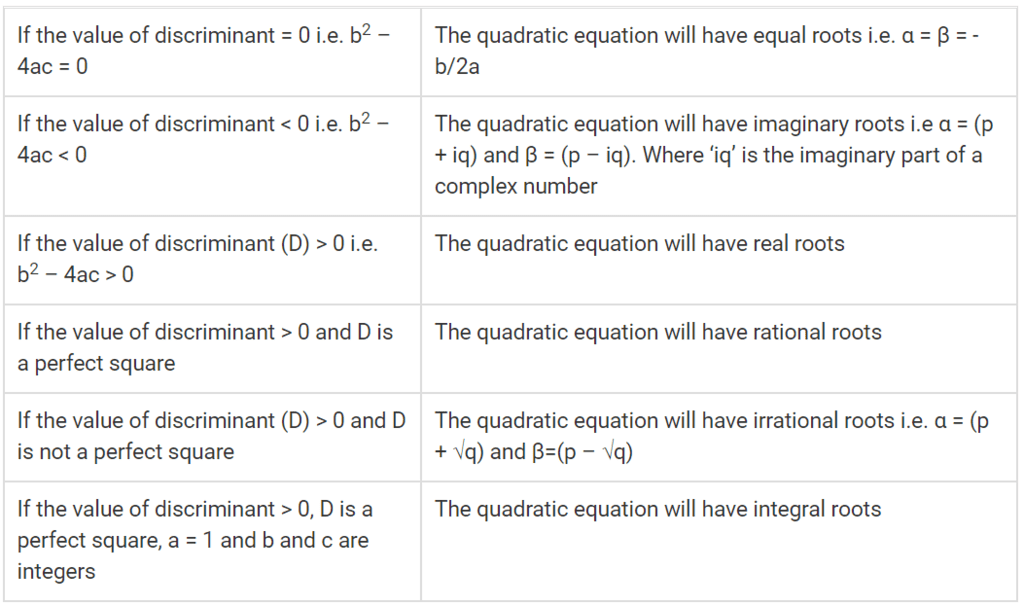Nature of Roots of Quadratic Equation

Question for Important Notes: Quadratic & Higher Order Equations
Try yourself:Roots of a quadratic equation are real when discriminant is ______.

### 2. Determination of Nature of Roots of a Quadratic Equation

Let's see how to determine nature of roots of a quadratic equation through these solved examples:

Example 1: Find the values of k for which the quadratic expression (x – a) (x – 10) + 1 = 0 has integral roots.

• The given equation can be rewritten as, x2 – (10 + k)x + 1 + 10k = 0.
D = b2 – 4ac = 100 + k2 + 20k – 40k = k2 – 20k + 96 = (k – 10)2 – 4
• The quadratic equation will have integral roots, if the value of discriminant > 0, D is a perfect square, a = 1 and b and c are integers.
i.e. (k – 10)2 – D = 4
• Since discriminant is a perfect square. Hence, the difference of two perfect square in R.H.S will be 4 only when D = 0 and (k – 10)2 = 4.
⇒ k – 10 = ± 2. Therefore, the values of k = 8 and 12.

Example 2: Find the values of k such that the equation p/(x + r) + q/(x – r) = k/2x has two equal roots.

• The given quadratic equation can be rewritten as:
[2p + 2q – k]x2 – 2r[p – q]x + r2k = 0
• For equal roots, the discriminant (D) = 0, i.e. b2 – 4ac = 0
Here, a = [ 2p + 2q – k ], b = – 2r [ p – q ] and c = r2k
[-2r (p – q)]2 – 4[(2p + 2q – k) (r2k)] = 0
r2(p – q)2 – r2k(2p + 2q – k) = 0
• Since r ≠ 0, therefore, (p – q)2 – k(2p + 2q – k) = 0
k2 – 2(p + q)k + (p – q)2
k = 2(p+q) ± √[4(p + q)2 – 4(p – q)]2/2 = -(p + q) ± √4pq
• ∴ The values of k = (p + q) ± 2√pq = (√p ± √q)2

3. Relationships between Coefficient and Roots of Quadratic Equation

If α and β are roots of a Quadratic Equation ax2 + bx + c then:

• α + β = -b/a
• αβ = c/a
• α – β = ±√[(α + β)2 – 4αβ]
• |α + β| = √D/|a|

The relationship between the roots and coefficient of a polynomial equation can be derived by simplifying the given polynomials and substituting the above results as shown below:

• α2β + β2α = αβ (α + β) = – bc/a2
• α2 + αβ + β2 = (α + β)2 – αβ = (b2 – ac)/a2
• α2 + β2 = (α – β)2 – 2αβ
• α2 – β2 = (α + β) (α – β)
• α3 + β3 = (α + β)3 + 3αβ(α + β)
• α3 – β3 = (α – β)3 + 3αβ(α – β)
• (α/β)2 + (β/α)2 = α4 + β42β2

Example 3: If the coefficient of x in the quadratic equation x2 + bx + c =0 was taken as 17 in place of 13, its roots were found to be -2 and -15. Find the roots of the original quadratic equation.

• Since there is no change in the coefficient of x2 and c, therefore, the product of zeros will remain the same for both equations.
• Therefore, the product of zeros (c) = -2 × -15 = 30,
Since, the original value of b is 13.
• ∴ Sum of zeros = -b/a = -13.
• Hence, the original quadratic equation is:
x2 – (Sum of Zeros)x + (Product of Zeros) = 0
x2 + 13x + 30 = 0
∴ (x + 10) (x + 3) = 0
• Therefore, the roots of the original quadratic equations are -3 and -10.

### 4. Quadratic Equations Having Common Roots

• Let β be the common root (solution) of quadratic equations a1x2 + b1x+c1 and a2x2+b2x+c2. This implies that a1β+ b1β + c= 0 and a2β+ b2β + c= 0.
• Now, Solving for β2 and β we will get:
β2/(b1c2 – b2c1) = -β/(a1c2 – a2c1) = 1/(a1b2 – a2b1) [using determinant method]
Therefore, β= (b1c2 – b2c1)/ (a1b2 – a2b1) . . . . . . . . . . . . . . . . (1)
And, β = (a2c1 – a1c2)/(a1b2 – a2b1) . . . . . . . . . . . . . . . . (2)
• On squaring equation (2) and equating it with equation (1) we get:
(a1b2 – a2b1)/(b1c2 – b2c1) = (a2c1 – a1c2)2
• Hence, it is the required condition for quadratic equations having one common root.
If both the roots of quadratic equations a1x2 + b1x + c1 and a2x2 + b2x + c2 are common then:
a1/a2 = b1/b2 = c1/c2
• If α is a repeated root, i.e., the two roots are α, α of the equation f(x) = 0, then α will be a root of the derived equation
f’(x) = 0 where f’(x) = df/dx
• If α is a repeated root common in f(x) = 0 and ϕ(x) = 0, then α is a common root both in f’(x) = 0 and ϕ ‘(x) = 0.

Let's see some solved examples to solve quadratic equations with common roots.

Example 4: For what value of k, both the quadratic equations 6x2 – 17x + 12 = 0 and 3x2 – 2x + k = 0 will have a common root.

• If one of the root of quadratic Equations a1x2 + b1x + c1 and a2x2 + b2x + c2 is common then: (a1b2 – a2b1)(b1c2 – b2c1) = (a2c1 – a1c2)2 . . . . . . . . . . . . . (1)
• Form the given quadratic Equations, a1 = 6, b1 = -17, c1 = 12, a2 = 3, b2 = -2 and c2 = k
• On substituting these values in equation (1), we will get:
[(6×-2) – (3×-17)] × [-17k – (-2×12)] = (3×12 – 6k)2
-663k + 936 = 1296 + 36k2 – 432k
36k2 + 231k + 360 = 0
12k2 + 125k + 120 = 0
(4k + 15) (3k + 8) = 0
• Therefore, the values of k are -154, -83.

Example 5: Find the values of k such that the Quadratic Equations x2 – 11x + k and x2 – 14x + 2k have a common factor.

• Let (x – α) be the common factor of quadratic Equations x2 – 11x + k and x2 – 14x + 2k Then x = α will satisfy the given quadratic equations.
• Therefore, α2 – 11α + k = 0 . . . . . . . . . . (1)
And, α2 – 14α + 2k = 0 . . . . . . . . . . . . (2)
• On Solving Equation (1) and Equation (2) we will get:
α2/(-22k + 14k) = -α/2k – k = 1/(-14 + 11)
Therefore, α= (-22k + 14k)/-3 = 8/3 . . . . . . . (3)
And, α = (2k – k)/(-14 + 11) = k/3 . . . . . . . . . . . (4)
• On Equating Equation (3) and Equation (4):
8/3 = (k/3)2
• Therefore, the value of k = 24.

## How to Solve Quadratic Equations?

There are two methods to solve a quadratic equation:

• Algebraic Method
• Graphical Method

### 1. Algebraic Method of Solving Quadratic Equations

General Form: ax2 + bx + c = 0;
⇒ x2 + bx/a + c/a = 0
⇒ (x + b/2a)2 = b2/4a2 – c/a
⇒ (x + b/2a)2 = (b2 – 4ac)/4a2
⇒ x + b/2a = ± (√b2 – 4ac)/2a
⇒ x = [-b ± √(b2 – 4ac)]/2a
b2  – 4ac = Discriminant (D)

α = (-b+√D)/2a

β = (-b – √D)/2a

α+β= -b/a, α.β = c/a
Therefore, the quadratic equation can be written as,
x2 – (α + β)x + (α.β) = 0.

Tips to Solve Equations reducible to Quadratic:

• To solve the equations of type ax4 + bx2 + c = 0, put x2 = y
• To solve a.p(x)+ b.p(x) + c = 0, put p(x) = y.
• To solve a.p(x) + b/p(x) + c = 0, put p(x) = y.
• To solve a(x2 + 1/x2) + b(x + 1/x) + c = 0,put x + 1/x = y and to solve a(x2 + 1/x2) + b(x – 1/x) + c = 0, put x – 1/x = y.
• To solve a reciprocal equation of the type ax4 + bx3 + cx2 + bx + a = 0, a ≠ 0, divide the equation by d2y/dx2 to obtain a(x+ 1/x2) + b(x + 1/x) + c = 0,and then put x + 1/x = y.
• To solve (x + a) (x + b) (x + c) (x + d) + k = 0 where a + b = c + d, put x2 +(a + b)x = y
• To solve an equation of type √(ax + b) = cx + d or √(ax2 + bx + c) = dx + e, square both the sides.
• To solve √(ax + b) ± √(cx + d) = e, transfer one of the radical to the other side and square both the sides. Keep the expression with radical sign on one side and transfer the remaining expression on the other side.

### 2. Graphical Solution of Quadratic Equation

• Consider a quadratic equation  ax2 + bx + c = 0, where a, b, and c are real and a ≠ 0. The expression can be further rewritten as:
a[(x + b/2a)2 + (D/4a2)]
• The above quadratic equation represents a parabola whose vertex is at P [-b/2a, -D/4a] and axis parallel to y-axis.
• In a quadratic equation, the value of ‘a’ determines whether the graph of a quadratic equation will be concave upwards (a > 0) or concave downwards (a < 0). The value of discriminant (b2 – 4ac) determines whether the graph of a quadratic equation will:
(i) Intersect the x-axis at two points i.e. b2 – 4ac > 0
(ii) Just touches the x-axis i.e. b2 – 4ac = 0
(iii) Never intersects the x-axis i.e. b2 – 4ac < 0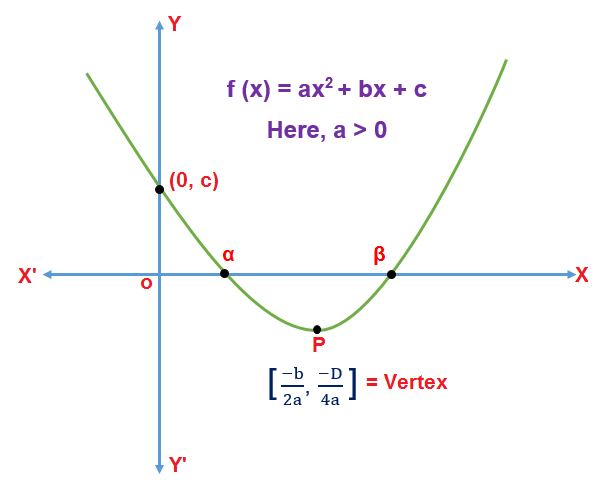The quadratic equation representing a parabola with vertex at P and axis parallel to the y-axis.
Case 1: When a > 0 and b2 – 4ac > 0

• The graph of a quadratic Equation will be concave upwards and will intersect the x-axis at two points α and β with α < β. The quadratic equation will have two real roots (α and β) and the curve will always lie above the x-axis.
• The quadratic function f(x) will be positive i.e. f(x) > 0, for the values of x lying in the interval (-∞, α) ∪ (β, ∞)
• The quadratic function f(x) will be Equal to zero i.e. f(x) = 0, if x = α or β
• The quadratic function f(x) will be negative i.e. f(x) < 0, for the values of x lying in the interval (α, β)

Case 2: When a > 0 and b2 – 4ac = 0

• The graph of a quadratic equation will be concave upwards and will touch x-axis at a point -b/2a.
• The quadratic equation will have two equal real roots i.e. α = β. The quadratic function f(x) will be positive i.e. 0 ≤ f(x), x ∈ R.

Case 3: When a > 0 and b2 – 4ac < 0

• The graph of a quadratic equation will be concave upwards and will not intersect the x-axis. The quadratic equation will have imaginary roots and the curve will always lie above the x-axis.
• The quadratic function f(x) will be positive i.e. f(x) > 0, x ∈ R.

Case 4: When a < 0 and  b2 – 4ac > 0

• The graph of a quadratic equation will be concave downwards and will intersect the x-axis at two points α and β with α < β. The quadratic equation will have two real roots (α and β) and the curve will always lie below the x-axis.
• The quadratic function f(x) will be positive i.e. f(x) > 0, for the values of x lying in the interval (α, β).
• The quadratic function f(x) will be equal to zero i.e. f(x) = 0, if x = α or β
• The quadratic function f(x) will be negative i.e. f(x) < 0 for the values of x lying in the interval (−∞, α) ∪ (β, ∞)

Case 5: When a < 0 and b2 – 4ac = 0

• The graph of a quadratic equation will be concave downwards and will touch x-axis at a point -b/2a.
• The quadratic equation will have equal real roots i.e. α = β. The quadratic function f(x) will be negative i.e. f(x) ≤ 0, x ∈ R.

Case 6: When a < 0 and b2 – 4ac < 0

• The graph of a quadratic equation will be concave downwards and will not intersect the x-axis.
• The quadratic equation will have imaginary roots and the curve will always lie below the x-axis. The quadratic function f(x) will be negative i.e. f(x) < 0, x ∈ R.

Consider a quadratic expression f(x) = ax2 + bx + c, where a ≠ 0 and a, b, and c are real. The quadratic expression can be further rewritten as f(x) = x2 + bax = ca.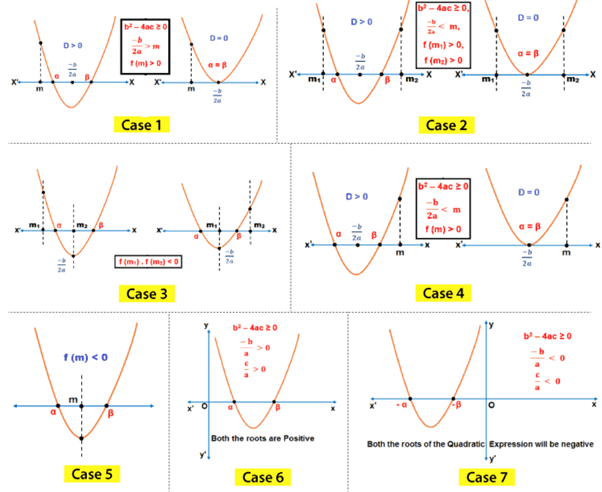Intervals in which the roots of Quadratic Equation lie

Case 1: Both the roots of a quadratic equation (α, β) are greater than any given number ‘m’ if,

• b2 – 4ac = (D) ≥ 0,
• -b/2a > m,
• f (m) > 0.

Case 2: Both the roots of a quadratic expression (α, β) are less than any given number ‘m’ if,

• b– 4ac = (D) ≥ 0,
• -b/2a < m,
• f(m) > 0.

Case 3: Both the roots of a quadratic expression (α, β) will lie in the given interval (m1, m2) if,

• b– 4ac = (D) ≥ 0,
• m1 < -b/2a > m2,
• f(m1) > 0,
• f(m2) > 0.

Case 4: If exactly one root of a quadratic equation (α, β) will lie in the given interval (m1, m2) if, f(m1).f(m2) < 0
Case 5: The given number ‘m’ will lie between the roots of a quadratic Equation α and β if, f(m) < 0
Case 6: The roots of a quadratic equation α and β will have an opposite sign if, f(0) < 0.
Case 7: Both the roots of a quadratic expression α and β are positive if,

• b2 – 4ac = (D) ≥ 0,
• α + β = -b/a > 0,
• αβ = c/a > 0.

Case 8: Both the roots of a quadratic expression α and β are negative if,

• b2 – 4ac = (D) ≥ 0,
• 2. α + β = -b/a < 0,
• 3. αβ = c/a < 0.

Example 6: Find the range of k for which 6 lies between the roots of the quadratic equation x2 + 2(k – 3)x + 9 = 0.

• 6 will lie between the roots of the quadratic expression f(x) = x+ 2(k – 3)x + 9 if,
f (6) < 0
i.e. 36 + 2 (k – 3) . 6 + 9 < 0,
= 36 + 12k – 36 + 9 < 0,
= k < -34
• Therefore, the range of k for which 6 lies between the roots of the given quadratic equation is:
k ∈ (-∞, -34)

### Maximum and Minimum Value of Quadratic Equation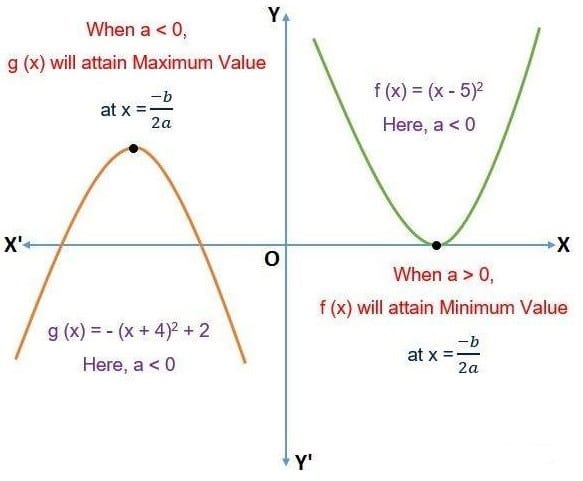Conditions for Minimum and Maximum Value of Quadratic Equation

• To find the minimum value of a quadratic equation we need to understand the nature of the graph of these equations for different values of ‘a’. The graph of the quadratic equation f(x) = ax2 + bx + c will be either concave upwards (a > 0) or concave downwards (a < 0) respectively.
• When the graph is concave upwards then its vertex determines the minimum value of a quadratic function f(x) and when it is concave downwards, its vertex determines the maximum values of a quadratic function f(x).

How to Find Maximum and Minimum Values of Quadratic Functions?

Case 1

• When a > 0, the absolute range of a quadratic expression is given by:
[-b2 – 4ac/4a, ∞) or [-D/4a, ∞)

Case 2

• When a < 0, the absolute range of a quadratic equation is given by:
(-∞, -D/4a]
• Also, the maximum and minimum values of a quadratic equation f(x) occurs at x = -b/2a.
• If the given quadratic equation is in the form f(x) = a(x – h)2 + m, Then the value of ‘m’ (vertex) gives us the minimum (when ‘a’ is negative) or maximum (when ‘a’ is positive) values of the given function.

Maximum and Minimum Values in Restricted Domain
Case 1

• -b/2a ∉ [m, n]
• f(x) = ∈ [ minimum {f(m), f(n)}, maximum {f(m), f(n)} ]

Case 2

• -b/2a ∈ [m, n]
• f(x) ∈ [minimum {f(m), f(n) – D/4a}, maximum {f(m), f(n), -D/4a}]

Example 7: Find the maximum or minimum value of quadratic equation -4(x – 2)+ 2.

• Since the value of ‘a’ is negative, therefore the given quadratic equation will have a maximum value.
• Hence, the maximum value of the quadratic equation -4(x – 2)2 + 2 is 2.

Example 8: Find the minimum and maximum values of quadratic equation f(x) = x2 – 12x + 11.

• Since a > 0, the maximum and minimum values of a quadratic expression is given by:
[(-b– 4ac)/4a, ∞) or [-D/4a, ∞)
• Therefore, the minimum value of f(x) is:
-(144 – 44)/4 at x = -(-12/2) = -25 at x = 6.
• The maximum value of f(x) is infinity. Therefore, the range of the given quadratic equation is [- 25, ∞).

### Descartes' Rule of Signs for Quadratic Polynomials

• This is a very famous rule that helps in getting an idea about the roots of a polynomial equation.
• The rule states that the number of positive real roots of Pn(x) = 0 cannot be more than the number of sign changes. Similarly, the number of negative roots cannot be more than the number of sign changes in Pn(-x).

Example 9: How many real roots does the equation 2x5 + 2x4 – 11x+ 9x2 – 4x + 2 = 0 will have?

• The given equation has 4 sign changes so it can have a maximum of 4 positive real roots.
• Now for f(-x), the equation has only one sign change i.e. f (-x) = -x5 + 2x4 + x3 + x2 + x + 2 = 0. Hence, the equation will have only one negative real root.

Example 10: How many real roots do the quadratic equation x+ 3|x| + 2 will have?

• Since the quadratic equation has no sign change for both f(x) and f(-x). Therefore, the equation will have no real root.
• Sign Convention of Quadratic Equation – ax2 + bx + c = 0
• The roots of quadratic equation are equal in magnitude but of opposite sign if b = 0 and ac < 0
• The root with greater magnitude is negative if the sign of a = sign of b × sign of c
• If a > 0, c < 0 or a > 0, c > 0; the roots of quadratic equation will have opposite sign
• If y = ax2 + bx + c is positive for all real values of x, a > 0 and D < 0
• If y = ax2 + bx + c is negative for all real values of x, a < 0 and D < 0

### Quadratic Equations in Two Variables

• The general form of a quadratic equation ax2 + 2hxy + by2 + 2gx + 2fy + c can be factorized into two linear factors as shown below,
ax2 + 2(hx + g)x + by2 + 2fy + c = 0  . . . .    (i)
• Using the quadratic formula in the above equation we get,
x = [2(hy + g) ± √{4(hy + g)2 – 4a(by2 + 2fy + c)}]/2a
ax + hy + g = ± √(h2y2  + g2 + 2ghy – aby2  – 2afy – ac) . . . . (ii)
• At this point, the expression (i) can be resolved into two linear factors if,
(h2 – ab)y2 + 2(gh – af)y + g2 – ac is a perfect square and h2 – ab > 0
But (h2 – ab)y2 + 2(gh – af)y + g2 – ac will be a perfect square if discriminant = 0
i.e. g2h2 + a2f2 – 2afgh – h2g2 + abg2 + ach2 – a2bc = 0 and h2 – ab > 0
abc + 2fgh – af2 – bg2 – ch2 = 0 and h2 – ab > 0
• This is the required condition. The condition that this expression may be resolved into two linear rational factors is:
abc + 2fgh – af2 – bg2 – ch2 = 0 and h2 – ab > 0
• This expression is called the discriminant of the above quadratic expression.

## Cubic Equation

•  A polynomial having degree three is known as a cubic polynomial or we can call it a cubic equation.
• Cubic equations have at least one real root and they can have up to 3 real roots.
• The roots of a cubic equation can be imaginary as well but at least 1 must be real.
ax3 + bx2 + cx + d = 0
⇒ Sum of the roots = - b/a
⇒ Sum of the product of the roots taken two at a time = c/a
⇒ Product of the roots = -d/a
• Example: Find the roots of the following cubic equation 2x3 + 3x2 – 11x – 6 = 0
Solution:
To find: Roots of the given equation.
This equation can not be solved using the factorization method, we will use the trial and error method to find one root.
f (1) = 2 + 3 – 11 – 6 ≠ 0
f (–1) = –2 + 3 + 11 – 6 ≠ 0
f (2) = 16 + 12 – 22 – 6 = 0
Value “2” makes the L.H.S equal to “0”. Hence two is one of the three roots.
Now we will use Synthetic Division Method to find the other two roots.
We divide our equation by (x-2) and the quotient will give us the other two roots. We divide our equation by (x-2) and the quotient will give us the other two roots.
Quotient : (2x2 + 7x + 3)
Factorising this quotient,
(2x+1) (x+3)
From here we get the values of x as,
x = -1/2 and x = -3
Answer: So, the three roots of the cubic equation are x = 2, x = -1/2 and x = -3

## Higher Degree Equations/ Biquadratic Equation

• A polynomial equation of degree four without the terms of degree three and one is known as a biquadratic equation.
• General Form: z4 + a0z2 + c = 0
Examples: (i) x4 +16x2 + 6 = 0
(ii) 2x4 + 14x2 + 3 = 0
• Biquadratic Equation ax4+bx3+cx2+dx+e = 0
⇒ Sum of the roots = - b/a
⇒ Sum of the product of the roots taken three at a time = c/a
⇒ Sum of the product of the roots taken two at a time = -d/a
⇒ Product of the roots = e/a

Let's see an example to solve Biquadratic Equations

Biquadratic polynomials can be easily solved by converting them into quadratic equations i.e. by replacing the variable ‘z’ by x2.

Example 11: Find the zeros of a biquadratic equation x– 3x2 + 2 = 0.

• Given f (x) = x– 3x2 + 2
• On substituting x2 = z in the given equation we get,
f(x) = z2 – 3z + 2 = 0
z2 – 2z – z + 2 = 0
z(z – 2) -1(z – 2) = 0
∴ z = 1 and z = 2
• Hence, x = ±√1 and x = ±√2 [Since, z = x²].

The document Quadratic & Higher Order Equations Important Notes | CSAT Preparation - UPSC is a part of the UPSC Course CSAT Preparation.
All you need of UPSC at this link: UPSC

## CSAT Preparation

197 videos|151 docs|200 tests

## FAQs on Quadratic & Higher Order Equations Important Notes - CSAT Preparation - UPSC

 1. What is a quadratic equation?A quadratic equation is a polynomial equation of the second degree, which can be written in the form ax^2 + bx + c = 0, where a, b, and c are constants and a ≠ 0.
 2. What are the roots of a quadratic equation?The roots of a quadratic equation are the values of x that satisfy the equation and make it equal to zero. A quadratic equation can have two, one, or no real roots, depending on the discriminant (b^2 - 4ac).
 3. How can we determine the nature of the roots of a quadratic equation?The nature of the roots of a quadratic equation can be determined by evaluating the discriminant. 1. If the discriminant is greater than 0, the equation has two distinct real roots. 2. If the discriminant is equal to 0, the equation has two equal real roots. 3. If the discriminant is less than 0, the equation has no real roots and the roots are complex conjugates.
 4. What are the important formulas for solving quadratic equations?The important formulas for solving quadratic equations are: 1. Quadratic Formula: x = (-b ± √(b^2 - 4ac)) / 2a 2. Sum of roots: (-b/a) 3. Product of roots: (c/a)
 5. How can we solve quadratic equations?Quadratic equations can be solved by using various methods such as factoring, completing the square, or by using the quadratic formula. The quadratic formula is the most general and reliable method to solve any quadratic equation.

## CSAT Preparation

197 videos|151 docs|200 testsExplore Courses for UPSC exam### How to Prepare for UPSC

Read our guide to prepare for UPSC which is created by Toppers & the best Teachers
Signup to see your scores go up within 7 days! Learn & Practice with 1000+ FREE Notes, Videos & Tests.
10M+ students study on EduRev
Track your progress, build streaks, highlight & save important lessons and more!
Related Searches

,

,

,

,

,

,

,

,

,

,

,

,

,

,

,

,

,

,

,

,

,

;